# NCERT Solutions Class 11 Chemistry Chapter 1 Some Basic Concepts Of

Ncert Solutions for class 11 subject Chemistry Chapter 1 Some Basic Concepts Of in pdf Best Free NCERT Solutions for class 1 to 12 in pdf NCERT Solutions, cbse board, Chemistry, ncert Solutions for Class 11 Chemistry, class 11 Chemistry ncert solutions, Some Basic Concepts Of , Class 11, ncert solutions chapter 1 Some Basic Concepts Of , class 11 Chemistry, class 11 Chemistry ncert solutions, Chemistry ncert solutions class 11, Ncert Solutions Class 11 Chemistry Chapter 1 Some Basic Concepts Of Chemistry

## Concept Chapter 1 Class 11 Chemistry Some Basic Concepts Of Ncert Solutions

Question 1. Calculate the molecular mass of the following : (i) H2O (ii) CO2 (iii) CH4
Method 1
(i)Molecular mass of H2O=2 × Atomic Mass of H+Atomic Mass of O=2+16=18 a.m.u
(ii)Molecular mass of CO2 =Atomic Mass of C+2 × Atomic Mass of O=12+32=44 a.m.u
(iii)Molecular mass of CH4 =Atomic Mass of C+4 × Atomic Mass of H=12+4=16 a.m.u
Method 2
(i) H2O:
The molecular mass of water, H2O
= (2 × Atomic mass of hydrogen) + (1 × Atomic mass of oxygen)
= [2(1.0084) + 1(16.00 u)]
= 2.016 u + 16.00 u
= 18.016
= 18.02 u
(ii) CO2:
The molecular mass of carbon dioxide, CO2
= (1 × Atomic mass of carbon) + (2 × Atomic mass of oxygen)
= [1(12.011 u) + 2 (16.00 u)]
= 12.011 u + 32.00 u
= 44.01 u
(iii) CH4:
The molecular mass of methane, CH4
= (1 × Atomic mass of carbon) + (4 × Atomic mass of hydrogen)
= [1(12.011 u) + 4 (1.008 u)]
= 12.011 u + 4.032 u
= 16.043 u

What is chemistry ?
Chemistry is the science of molecules and their transformations. Chemistry is called the science of atoms and molecules. Chemistry is the branch of science that studies the composition, properties and interaction of matter.

what is the importance of chemistry?
(1) Chemistry plays an important role in meeting human needs for food, health care products and other materials
(2) Production of a variety of fertilizers, improved varieties of pesticides and insecticides
(3) Preparation of drugs isolated from plant and animal sources or prepared by synthetic methods.
(4) Design and synthesize new materials having specific magnetic, electric and optical properties.
(5) Production of superconducting ceramics, conducting polymers, optical fibres and large scale miniaturization of solid state devices.

What is matter ?
Anything which has mass and occupies space is called matter. for example, book, pen, pencil, water, air, all living beings etc. are composed of matter.
matter can exist in three physical states viz. solid, liquid and gas.

Solid :
Solids have definite volume and definite shape.
Reason In solids, these particles are held very close to each other in an orderly fashion and there is not much freedom of movement.
Liquid
Liquids have definite volume but not the definite shape. They take the shape of the container in which they are placed.
Reason In liquids, the particles are close to each other but they can move around
Gas:
Gases have neither definite volume nor definite shape. They completely occupy the container in which they are placed.
Reason :in gases, the particles are far apart as compared to those present in solid or liquid states and their movement is easy and fast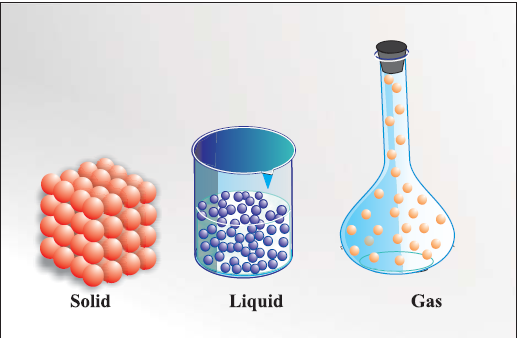Classification of matter:
Matter can be classified as mixtures or pure substances.
Mixture : A mixture contains two or more substances present in it (in any ratio) which are called its components.
For example, sugar solution in water, air, tea etc.
Mixtures are two types
heterogeneous mixtures : the components completely mix with each other and its composition is uniform throughout.
Examples Sugar solution, air
heterogeneous mixtures: the composition is not uniform throughout and sometimes the different components can be observed.
Example: mixtures of salt and sugar
What are the method of separation of component of mixture?
components of a mixture can be separated using physical methods such as simple hand picking, filtration, crystallisation, distillation etc

Pure substances. They have fixed composition . constituents components of pure substances cannot be separated by simple physical methods.
Examples Copper, silver, gold, water, glucose etc
Pure substances can be further classified into elements and compounds
Elements: An element consists of only one type of particles. These particles may be atoms or molecules.
Examples: Sodium, copper, silver, hydrogen, oxygen etc.
Compound: When two or more atoms of different elements combine, the molecule of a compound is obtained. the atoms of different elements are present in a compound in a fixed and definite ratio and this ratio is characteristic of a particular compound. the properties of a compound are different from those of its constituent elements. Constituent particles can be separated by chemical methods.
The examples of some compounds are water, ammonia, carbon dioxide, sugar etc
What are the physical properties of the matter?
Physical properties are those properties which can be measured or observed without changing the identity or the composition of the substance.
Examples: colour, odour, melting point,boiling point, density etc
What are the chemical properties?
Chemical properties are those properties which require a chemical changes to occur
Examples: acidity or basicity, combustibility etc.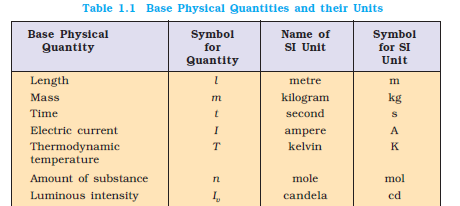1 mol : The mole is the amount of substance of a system which contains as many elementary
entities as there are atoms in 0.012 kilogram of carbon-12; its symbol is “mol”.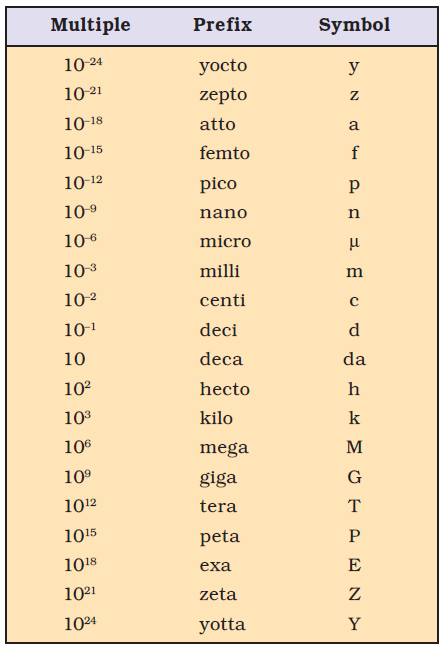Pico ,nano, micro , mili ,deci,centi ,deca, hecta, kilo and mega is important for exams and numerical problems

Scientific notation
It is exponential notation in which any number can be represented in the form N × 10n where n is an exponent having positive or negative values and N can vary between 1 to 10.
Example
we can write 232.508 as 2.32508 x 102 in scientific notation.
Teach addition , subtraction, multiplication and ision of exponential notations

Significant Figures
(1) Zeros at the end or right of a number are significant provided they are on the right side of the decimal point. Otherwise, the zeros are not significant. For example, 0.200 g has three significant figures. But100 has only one significant figure.
(2) Zeros preceding to first non-zero digit are not significant. Such zero indicates the position of decimal point.
Example:
0.03 has one significant figure
0.0052 has two significant figures.

(3) When numbers are written in scientific notation, the number of digits between 1 and 10 gives the number of significant figures
Examples:
4.01×102has three significant figures,
8.256 × 10–3has four significant figures.
(4)Zeros between two non-zero digits are significant. Thus, 2.005 has four significant figures.

Addition and Subtraction of Significant Figures
The result cannot have more digits to the right of the decimal point than either of the original numbers.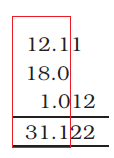Here, 18.0 has only one digit after the decimal point and the result should be reported only up to one digit after the decimal point which is 31.1.

Multiplication and Division of Significant Figures
In these operations, the result must be reported with no more significant figures as are there in the measurement with the few significant figures.
2.5×1.25 = 3.125
Since 2.5 has two significant figures, the result should not have more than two significant figures.
Rounding off the numbers
· If the rightmost digit to be removed is more than 5, the preceding number is increased by one. for example 1.386 If we have to remove 6, we have to round it to 1.39
· If the rightmost digit to be removed is less than 5, the preceding number is not changed. For example, 4.334 if 4 is to be removed, then the result is rounded upto 4.33.
· If the rightmost digit to be removed is 5, then the preceding number is not changed if it is an even number but it is increased by one if it is an odd number. For example, if 6.35 is to be rounded by removing 5, we have to increase 3 to 4 giving 6.4 as the result. However, if 6.25 is to be rounded off it is rounded off to 6.2.
Dimensional Analysis
Often while calculating, there is a need to convert units from one system to other. The method used to accomplish this is called factor label method or unit factor method or dimensional analysis.

LAWS OF CHEMICAL COMBINATIONS:

Law of Conservation of Mass
It states that matter can neither be created nor destroyed.
Law of Definite Proportions
It stated that a given compound always contains exactly the same proportion of elements by weight.
Example : Oxygen and hydrogen in water always bear ratio of 16:2 or 8:1 by mass
Law of Multiple Proportions
According to this law, if two elements can combine to form more than one compound, the masses of one element that combine with a fixed mass of the other element, are in the ratio of small whole numbers.
Example
Hydrogen + Oxygen → Water
2g 16g 18g
Hydrogen + Oxygen → Hydrogen Peroxide
2g 32g 34g
Here, the masses of oxygen (i.e. 16 g and 32 g) which combine with a fixed mass of hydrogen (2g) bear a simple ratio, i.e. 16:32 or 1: 2.
Gay Lussac’s Law of Gaseous Volumes
when gases combine or are produced in a chemical reaction they do so in a simple ratio by volume
provided all gases are at same temperature and pressure.
Example
Hydrogen + Oxygen → Water
100 mL 50 mL 100 mL
Thus, the volumes of hydrogen and oxygen which combine together (i.e. 100 mL and 50 mL) bear a simple ratio of 2:1
Avogadro proposed that equal volumes of gases at the same temperature and pressure should contain
equal number of molecules
Example .
Hydrogen + Oxygen → Water
we see that two volumes of hydrogen combine with one volume of oxygen to give two volumes of water without leaving any unreacted oxygen.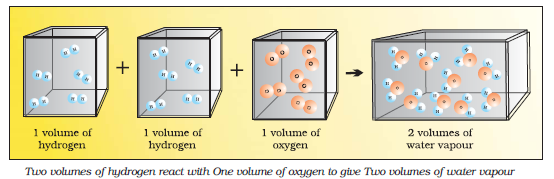DALTON’S ATOMIC THEORY
1. Matter consists of inisible atoms.
2. All the atoms of a given element have identical properties including identical mass. Atoms of different elements differ in mass.
3. Compounds are formed when atoms of different elements combine in a fixed ratio.
4. Chemical reactions involve reorganization of atoms. These are neither created nor destroyed in a chemical reaction.
5.Dalton’s theory could explain the laws of chemical combination.
amu: One atomic mass unit is defined as a mass exactly equal to one twelfth the mass of one carbon - 12 atom.
1 amu = 1.66056×10–24 g
Average Atomic Mass
Many naturally occurring elements exist as more than one isotope. When we take into account the existence of these isotopes and their relative abundance (per cent occurrence),
Example < style="margin-left:25px">Carbon has three isotopes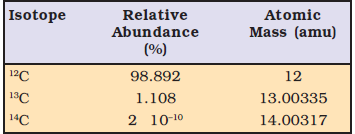Average mass
= (0.98892) (12 u) + ( 0.01108) (13.00335 u) + (2 × 10–12) (14.00317 u)
= 12.011 u
Molecular Mass
Molecular mass is the sum of atomic masses of the elements present in a molecule. It is obtained by multiplying the atomic mass of each element by the number of its atoms and adding them together.
Example
Molecular mass of methane,(CH4) = (12.011 u) + 4 (1.008 u) = 16.043 u
Formula mass
The formula such as NaCl is used to calculate the formula mass instead of molecular mass as in the solid state sodium chloride does not exist as a single entity.
Thus, formula mass of sodium chloride= atomic mass of sodium + atomic mass of chlorine
= 23.0 u + 35.5 u = 58.5 u
MOLE CONCEPT AND MOLAR MASSES
One mole is the amount of a substance that contains as many particles or entities as there are atoms in exactly 12 g (or 0.012 kg) of the 12C isotope.
The mass of one mole of a substance in grams is called its molar mass.
Empirical Formula for Molecular Formula

An empirical formula represents the simplest whole number ratio of various atoms present in a compound whereas the molecular formula shows the exact number of different types of atoms present in a molecule of a
compound.
Example
Molecular formula of glucose is C6H12O6 and there empirical formula will CH2O
STOICHIOMETRY AND STOICHIOMETRIC CALCULATIONS
Stoichiometry, deals with the calculation of masses (sometimes volumes also) of the reactants and the products involved in a chemical reaction. Before understanding how to calculate the amounts of reactants required or those produced in a chemical reaction,
Example
Let us consider the combustion of methane.
A balanced equation for this reaction is as given below :
CH4 (g) + 2O2 (g) → CO2 (g) + 2 H2O (g)
Thus, according to the above chemical reaction, we get
< class="fontx" style="margin-left:25px"> · Phase of reactant and product
· The coefficients 2 for O2 and H2O are called stoichiometric coefficients.
· One mole of CH4(g) reacts with two moles of O2(g) to give one mole of CO2(g) and two moles of H2O(g)
· One molecule of CH4(g) reacts with 2 molecules of O2(g) to give one molecule of CO2(g) and 2 molecules of H2O(g)
· 22.4 L of CH4(g) reacts with 44.8 L of O2 (g) to give 22.4 L of CO2 (g) and 44.8 L of H2O(g)
· 16 g of CH4 (g) reacts with 2×32 g of O2 (g) to give 44 g of CO2 (g) and 2×18 g of H2O (g).
Limiting Reagent
The reactant which is present in the lesser amount gets consumed after sometime and after that no further
reaction takes place whatever be the amount of the other reactant present is called limiting reagent. Or the reactant which gets consumed, limits the amount of product formed and is called the limiting reagent.
Mass per cent or weight per cent (w/w %) :
Mass % of an element = molar mass of that element x 100 / molar mass of compound
Mass % of a component = mass of component x 100 / total mass of the solution
Mole Fraction
It is the ratio of number of moles of a particular component to the total number of moles of the solution.
Mathematically
Mole fraction of component A = number of moles of component A / total number of moles
Molarity
Molarity is denoted by M. It is defined as the number of moles of the solute present in 1 litre of the solution.
Mathematically
Molarity (M) = No. of moles of solute / volume of solution in liter
Molality
Molality is denoted by m. It is defined as the number of moles of solute present in 1 kg of solvent.
Mathematically
Molality (m) = No. of mole of solute /Mass of solvent in Kg

## Exercise Chapter 1 Class 11 Chemistry Some Basic Concepts Of Ncert Solutions

(i)

Reaction of combustion of methane is

CH4 + 2O2 → 2H2O + CO2

According to the balanced equation, 1 mole of carbon burns with 1 mole of dioxygen and produce 2 mol of water and 1 mole of carbon dioxide.

Hence, Answer will 1 mol of CO2 will produced

(i) H2O

The molecular mass of water, H2O

= (2 × Atomic mass of hydrogen) + (1 × Atomic mass of oxygen)

Use Appendix II of NCERT book at page 232

= [2(1.0079) + 1(16.00)]

= 2.016 + 16.00

= 18.016

Approximate the value

= 18.02 amu

(ii) CO2

The molecular mass of carbon dioxide, CO2

= (1 × Atomic mass of carbon) + (2 × Atomic mass of oxygen)

Use Appendix II of NCERT book at page 232

= [1(12.01 ) + 2 (16.00 )]

= 12.01 + 32.00

= 44.01 amu

(iii) CH4

The molecular mass of methane, CH4

= (1 × Atomic mass of carbon) + (4 × Atomic mass of hydrogen)

Use Appendix II of NCERT book at page 232

= [1(12.01) + 4 (1.0079 )]

= 12.01 + 4.032

= 16.04 amu

Mass % of iron = 69.9 % [Given]

Mass % of oxygen = 30.1 % [Given]

 Element Atomic mass Mass % Mass % / atomic mass Fe 55.85 69.9 69.9/55.85 = 1.25 O 16.00 30.1 30.1/16.00 = 1.88

Fe : O = 1.25 : 1.88

Convert in simple ratio we get

Fe : O = 2 : 3

Hence, the empirical formula of the iron oxide is Fe2O3.

$$Mass\;\% \;of\;an\;element\; = \;{{molar\;mass\;of\;component} \over {molar\;mass\;of\;compound}}\; \times \;100$$
The molecular formula of compound (Na2SO4).is
Use Appendix II of NCERT book at page 232
= [(2 × 22.99) + (32.06) + 4 (16.00)]
= 142.04 g
Molar mass of 2Na = 2 × 22.99 = 45.98 amu
Molar mass of 4O = 4 × 16.00 = 64.00 amu
Plug the values in above formula
Mass percent of sodium: = 45.98 ×100 /142.04 = 32.37%
Mass percent of sulphur: = 32.06 ×100 /142.04 = 22.57%
Mass percent of oxygen: = 64.00 ×100 /142.04 = 45.06%

(ii) In this part mass of two reactants are given so we need to find the limiting reagent first

Atomic mass of C = 12 amu

Molecular mass of O2 = 2 × 16.00 = 32 amu

According to Stoichiometry of the reaction

12 g C will react with 32 g of O2

But we have only 16 g O2

Given mass of O2 (16 g) < required mass of O2 (32g)

Hence O2 is limiting reagent and product is always calculated with the help of mass of limiting reagent

Molecular mass of CO2 = 12 + 2× 16 = 44 amu

From the reaction

32 g of O2 produce = 44 g CO2

1 g of O2 Produce = 44/32 g CO2

16 g O2 will produce = 44 × 16 / 32 = 22 g CO2

(iii) In this part mass of two reactants are given so we need to find the limiting reagent first

Atomic mass of C = 12 amu

Molecular mass of O2 = 2 × 16.00 = 32 amu

2 mol of C = 2 x 12 = 24 g

According to Stoichiometry of the reaction

12 g C will react with = 32 g of O2

1 g of C will react with = 32/ 12 g O2

24 g of C will react with = 24 × 32 / 12 = 64 g O2

But we have only 16 g O2

Given mass of O2 (16 g) < required mass of O2 (64g)

Hence O2 is limiting reagent and product is always calculated with the help of mass of limiting reagent

Molecular mass of CO2 = 12 + 2× 16 = 44 amu

From the reaction

32 g of O2 produce = 44 g CO2

1 g of O2 Produce = 44/32 g CO2

16 g O2 will produce = 44 × 16 / 32 = 22 g CO2

Given that

0.375 Molar aqueous solution

Molar represent molarity of the solution

Hence given molarity of solution will 0.375M

Formula of molarity

Molarity (M) = No. of moles of solute / volume of solution in liter

Volume in liter = 500 ml = 0.5 liter (1000 ml = 1 liter)

Plug the values in above formula we get

Number of moles = 0.375 × 0.5 = 0.1875 mol

Use formula

Number of moles = mass / molar mass

Molar mass of sodium acetate = 82.0245 g mole-1 (Given)

Plug above values in formula we get

Mass of sodium acetate = (82.0245 g mol-1) (0.1875 mole) = 15.38 g

Given that Mass percent of nitric acid in the sample = 69 %

When mass % is given always take total mass = 100 g

Mass of nitric acid = 69% of 100 g = 69 g

Molar mass of nitric acid (HNO3)

= {1 + 14 + 3(16)} g mol-1 = 1 + 14 + 48 = 63 g mol-1

Use formula density = mass / volume

Given that density = 1.41 g ml-1

Total mass = 100 g

Plug the values in above formula

Volume of nitric acid = 100/1.41 = 70.92 ml

Concentration in moles per litre = number of moles / volume in liter …(1)

Number of moles = mass / molar mass …(2)

Molar mass of nitric acid (HNO3) = 1×1 + 14 + 3 × 16 = 63 amu

Plug the value in equation (2) we get

Number of moles of HNO3 = 69/63 = 1.095

Volume in liter = 70.92 ml = 0.07092 liter (ide by 1000 to convert in liter)

Plug the value in equation (1), we get

Concentration in moles per liter = 1.095/ 0.07092 = 15.44 mol / liter

1 mole of CuSO4 contains 1 mole of copper.

Molar mass of CuSO4

= Atomic mass of [Cu × 1 + S × 1 + O × 4 ]

=(63.5) + (32.00) + 4(16.0) = 63.5 + 32.0 + 64.0

= 159.5 g

Here molar mass of Cu is 159.5 g so that 159.5 of CuSO4 will produce 1 mole of Cu and mass of 1 mole of Cu is 63.5 g of copper.

So 100 g of CuSO4 will produce = $\frac{{63.5 \times 100}}{{159.5}} = 39.8g$

Answer : Amount of copper obtained from 100 g CuSO4 = 39.8 g

Mass % of iron = 69.9 % [Given]

Mass % of oxygen = 30.1 % [Given]

 Element Atomic mass Mass % Mass % / atomic mass Fe 55.85 69.9 69.9/55.85 = 1.25 O 16.00 30.1 30.1/16.00 = 1.88

Fe : O = 1.25 : 1.88

Convert in simple ratio we get

Fe : O = 2 : 3

The empirical formula of the iron oxide is Fe2O3.

N = Molar mass / Empirical mass …(1)

Empirical formula mass of Fe2O3 = [2×55.85 + 3×16.00] g = 159.70 g

Given that Molar mass of Fe2O3 = 159.69 g

Plug the values in equation (1), we get

N = 159.69/159.70 = 1

Molecular formula = Empirical formula × n = Fe2O3 × 1 = Fe2O3

Hence molecular formula is also Fe2O3

Molecular formula of a compound is obtained by multiplying the empirical formula with n. Thus, the empirical formula of the given oxide is Fe2O3 and n is 1.

Hence, the molecular formula of the oxide is Fe2O3.

If C1 and C2 is % abundance and M1 and M2 is molar mass of element of an isotopes respectively than

The average atomic mass of isotopes = (C1 ×M1 + C2×M2)/100

Plug the values we get

The average atomic mass of chlorine = (75.77 × 34.9689 + 24.23 × 36.9659)/100

= 35.4527 u

(i) There are two atoms of Carbon in each C2H6 molecules hence 1 mole of C2H6 will have 2 moles of carbon atoms. So that Number of moles of carbon atoms in 3 moles of C2H6 = 2 × 3 = 6 moles

(ii) There are six atoms of hydrogen in each molecules of C2H6 hence 1 mole of C2H6 will have 6 moles of hydrogen atoms. So that Number of moles of carbon atoms in 3 moles of C2H6 = 3 × 6 = 18 moles

(iii) Each mole of any substance has 6.023 × 1023 particles so that 3 mol of C2H6
will have = 3 × 6.023 × 1023 = 18.069 × 1023 molecules.

### NCERT Books Free Pdf Download for Class 5, 6, 7, 8, 9, 10 , 11, 12 Hindi and English Medium

 Mathematics Biology Psychology Chemistry English Economics Sociology Hindi Business Studies Geography Science Political Science Statistics Physics Accountancy